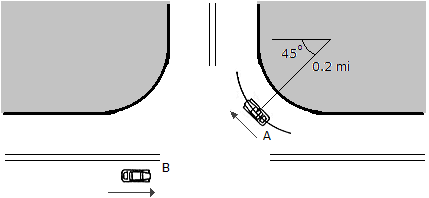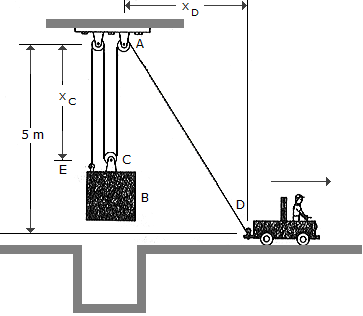# Engineering Mechanics - Kinematics of Particle (KOP)

### Exercise :: Kinematics of Particle (KOP) - General Questions

• Kinematics of Particle (KOP) - General Questions
26.

A boat is traveling along a circular path having a radius of 20 m. Determine the magnitude of the boat's acceleration if at a given instant the boat's speed is v = 5 m/s and the rate of increase in speed is v = 2 m/s2.

 A. a = 2.00 m/s2 B. a = 2.36 m/s2 C. a = 1.25 m/s2 D. a = 12.50 m/s2

Explanation:

No answer description available for this question. Let us discuss.

27.As the instant shown, cars A and B are traveling at speeds of 20 mi/h and 45 mi/h, respectively. If B is acceleration at 1600 mi/h2 while A maintains a constant speed, determine the magnitudes of the velocity and acceleration of A with respect to B.

 A. vA/B = 33.9 mi/h, aA/B = 1600 mi/h2 B. vA/B = 60.8 mi/h, aA/B = 1600 mi/h2 C. vA/B = 33.9 mi/h, aA/B = 1426 mi/h2 D. vA/B = 60.8 mi/h, aA/B = 1426 mi/h2

Explanation:

No answer description available for this question. Let us discuss.

28.The block B is suspended from a cable that is attached to the block at E, wraps around three pulleys, and is tied to the back of a truck. If the truck starts from rest when xD is zero, and moves forward with a constant acceleration of aD = 2 m/s2, determine the speed of the block at the instant xD = 3 m.

 A. vB = 0.1715 m/s B. vB = 1.155 m/s C. vB = 0.594 m/s D. vB = 0.515 m/s

Explanation:

No answer description available for this question. Let us discuss.

29.

A train travels along a horizontal circular curve that has a radius of 200 m. If the speed of the train is uniformly increased from 30 km/h to 45 km/h in 5 s, determine the magnitude of the acceleration at the instant the speed of the train is 40 km/h.

 A. a = 0.617 m/s2 B. a = 1.037 m/s2 C. a = 1.451 m/s2 D. a = 0.833 m/s2

Explanation:

No answer description available for this question. Let us discuss.

30.

A fly traveling horizontally at a constant speed enters the open window of a train and leaves through the opposite window 3 m away 0.75 s later. If the fly travels perpendicular to the train's motion as seen from an observer on the ground, and the train is traveling at 3 m/s, determine the speed of the fly as observed by a passenger on the train.

 A. vF/T = 2.65 m/s B. vF/T = 5.00 m/s C. vF/T = 1.000 m/s D. vF/T = 7.00 m/s